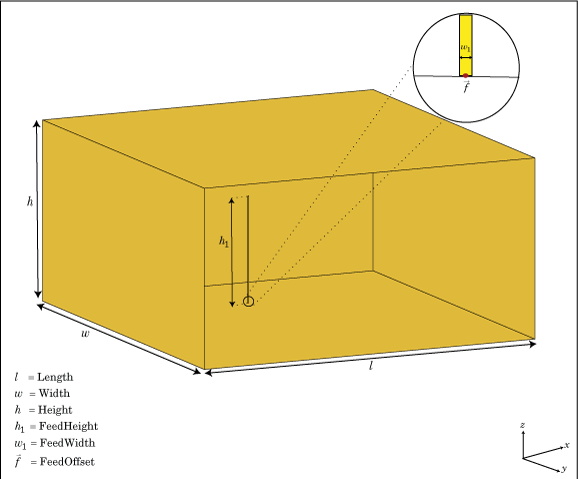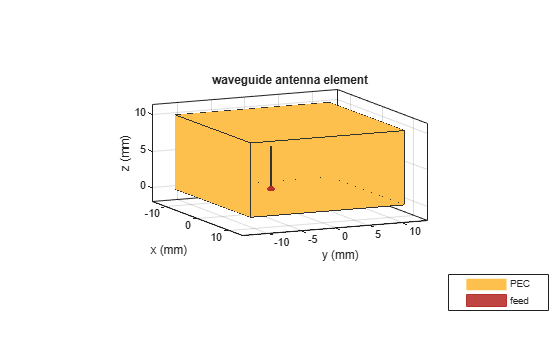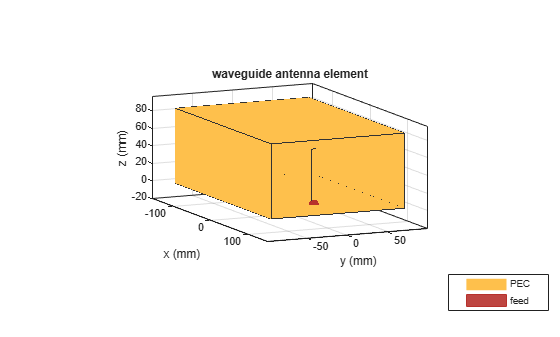# waveguide

Create rectangular waveguide

## Description

The `waveguide` object is an open-ended rectangular waveguide. The default rectangular waveguide is the WR-90 and functions in the X-band. The X-band has a cutoff frequency of 6.5 GHz and ranges from 8.2 GHz to 12.5 GHz.## Creation

### Syntax

``wg = waveguide``
``wg = waveguide(Name,Value)``

### Description

example

``` `wg = waveguide` creates an open-ended rectangular waveguide.```

example

``` `wg = waveguide(Name,Value)` creates a rectangular waveguide with additional properties specified by one, or more name-value pair arguments. `Name` is the property name and `Value` is the corresponding value. You can specify several name-value pair arguments in any order as `Name1,Value1,...,NameN,ValueN`. Properties not specified retain their default values.```

## Properties

expand all

Height of feed, specified as a scalar in meters. By default, the feed height is chosen for an operating frequency of 12.5 GHz.

Example: `'FeedHeight',0.0050`

Data Types: `double`

Width of feed, specified as a scalar in meters.

Example: `'FeedWidth',5e-05`

Data Types: `double`

Rectangular waveguide length, specified as a scalar in meters. By default, the waveguide length is 1λ, where:

`$\lambda =c/f$`

• `c` = speed of light, `299792458` m/s

• `f` = operating frequency of the waveguide

Example: `'Length',0.09`

Data Types: `double`

Rectangular waveguide width, specified as a scalar in meters.

Example: `'Width',0.05`

Data Types: `double`

Rectangular waveguide height, specified as a scalar in meters.

Example: `'Height',0.0200`

Data Types: `double`

Signed distance of feedpoint from center of ground plane, specified as a two-element vector in meters. By default, the feed is at an offset of λ/4 from the shortened end on the xy- plane.

Example: `'FeedOffset',[–0.0070 0.01]`

Data Types: `double`

Type of the metal used as a conductor, specified as a metal material object. You can choose any metal from the `MetalCatalog` or specify a metal of your choice. For more information, see `metal`. For more information on metal conductor meshing, see Meshing.

Example: ```m = metal('Copper'); 'Conductor',m```

Example: ```m = metal('Copper'); ant.Conductor = m```

Lumped elements added to the antenna feed, specified as a lumped element object. For more information, see `lumpedElement`.

Example: `'Load',lumpedelement`. `lumpedelement` is the object for the load created using `lumpedElement`.

Example: ```wg.Load = lumpedElement('Impedance',75)```

Tilt angle of the antenna, specified as a scalar or vector with each element unit in degrees. For more information, see Rotate Antennas and Arrays.

Example: `Tilt=90`

Example: `Tilt=[90 90]`,`TiltAxis=[0 1 0;0 1 1]` tilts the antenna at 90 degrees about the two axes defined by the vectors.

Note

The `wireStack` antenna object only accepts the dot method to change its properties.

Data Types: `double`

Tilt axis of the antenna, specified as:

• Three-element vector of Cartesian coordinates in meters. In this case, each coordinate in the vector starts at the origin and lies along the specified points on the X-, Y-, and Z-axes.

• Two points in space, each specified as three-element vectors of Cartesian coordinates. In this case, the antenna rotates around the line joining the two points in space.

• A string input describing simple rotations around one of the principal axes, 'X', 'Y', or 'Z'.

Example: `TiltAxis=[0 1 0]`

Example: `TiltAxis=[0 0 0;0 1 0]`

Example: `TiltAxis = 'Z'`

Data Types: `double`

## Object Functions

 `show` Display antenna, array structures or shapes `info` Display information about antenna or array `axialRatio` Axial ratio of antenna `beamwidth` Beamwidth of antenna `charge` Charge distribution on antenna or array surface `current` Current distribution on antenna or array surface `design` Design prototype antenna or arrays for resonance around specified frequency `efficiency` Radiation efficiency of antenna `EHfields` Electric and magnetic fields of antennas; Embedded electric and magnetic fields of antenna element in arrays `impedance` Input impedance of antenna; scan impedance of array `mesh` Mesh properties of metal, dielectric antenna, or array structure `meshconfig` Change mesh mode of antenna structure `optimize` Optimize antenna or array using SADEA optimizer `pattern` Radiation pattern and phase of antenna or array; Embedded pattern of antenna element in array `patternAzimuth` Azimuth pattern of antenna or array `patternElevation` Elevation pattern of antenna or array `rcs` Calculate and plot radar cross section (RCS) of platform, antenna, or array `returnLoss` Return loss of antenna; scan return loss of array `sparameters` Calculate S-parameter for antenna and antenna array objects `vswr` Voltage standing wave ratio of antenna

## Examples

collapse all

Create a rectangular waveguide using default dimensions. Display the waveguide.

`wg = waveguide`
```wg = waveguide with properties: Length: 0.0240 Width: 0.0229 Height: 0.0102 FeedWidth: 6.0000e-05 FeedHeight: 0.0060 FeedOffset: [-0.0060 0] Conductor: [1x1 metal] Tilt: 0 TiltAxis: [1 0 0] Load: [1x1 lumpedElement] ```
`show(wg)`Create a WR-650 rectangular waveguide and display it.

```wg = waveguide('Length',0.254,'Width',0.1651,'Height',0.0855,... 'FeedHeight',0.0635,'FeedWidth',0.00508,'FeedOffset',[0.0635 0]); show(wg)```Plot the radiation pattern of this waveguide at 1.5 GHz.

```figure pattern(wg,1.5e9)```Balanis, Constantine A.Antenna Theory. Analysis and Design. 3rd Ed. New York: John Wiley and Sons, 2005.